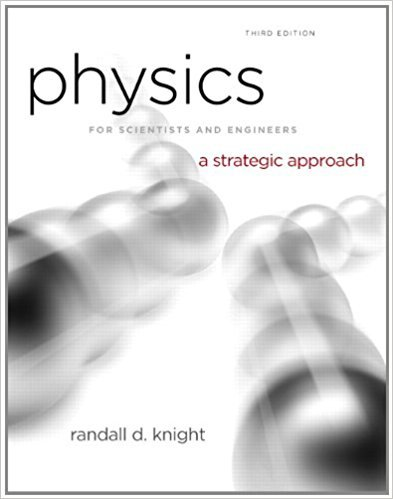×
Get Full Access to Physics For Scientists And Engineers: A Strategic Approach With Modern Physics - 3 Edition - Chapter 11 - Problem 70p
Get Full Access to Physics For Scientists And Engineers: A Strategic Approach With Modern Physics - 3 Edition - Chapter 11 - Problem 70p

×

# Solution: In are given the equation(s) used to solve aISBN: 9780321740908 69

## Solution for problem 70P Chapter 11

Physics for Scientists and Engineers: A Strategic Approach with Modern Physics | 3rd Edition

• Textbook Solutions
• 2901 Step-by-step solutions solved by professors and subject experts
• Get 24/7 help from StudySoup virtual teaching assistantsPhysics for Scientists and Engineers: A Strategic Approach with Modern Physics | 3rd Edition

4 5 1 338 Reviews
18
3
Problem 70P

Problem 70P

In Problem you are given the equation(s) used to solve a problem. For each of these, you are to

a. Write a realistic problem for which this is the correct equation(s).

b. Draw a pictorial representation.

c. Finish the solution of the problem.

Fpush − (0.20) (30 kg) (9.8 m/s2) = 0

75W=Fpushv

Step-by-Step Solution:

Solution 70P

Step 1 of 4:

In this question, we need to write realistic question to which the given equation are the solution

In part b, we need to pictorial representation of the question mentioned

In part c, we need to find solution for the given equations

Equations givenPart a:

Step 2 of 4

Step 3 of 4

##### ISBN: 9780321740908

Unlock Textbook Solution